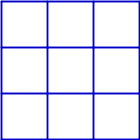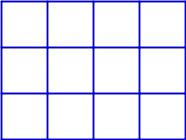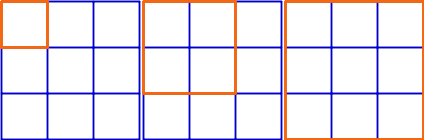# A Puzzle Involving Squares It is simpler than you think, but it took The Great Radar Oven ages.

## Problem

### Part 1

Look at this 3 by 3 grid. How many squares are there?Figure 1: Hint: It's not 9

### Part 2

How would you get the squares for a 100 by 100 grid without counting them?

Find a general equation to model this.

### Part 3

How would the equation change if the grid was not square, but rectangular instead?### Extensions

#### Cube

Using the prerious answers, what steps would be needed to find the square faces (both internal and external) of a 3D cube-shaped grid?

## Solutions

### Part 1

14

That being:

• 9 squares of size 1 by 1
• 4 squares of size 2 by 2
• 1 square of size 3 by 3### Part 2

$f(x) = x^2 + (x-1)^{2} + (x-2)^{2} + ... + (x-x)^{2}$

$x \geq 0$

Where $$x$$ is the side length of the square grid.

For a 3 by 3 grid:

$f(3) = 3^2 + (3-1)^{2} + (3-2)^{2} + (3-3)^{2} = 9 + 4 + 1 + 0 = 14$

### Part 3

$g(x,y) = xy + (x-1)(y-1) + (x-2)(y-2) + ... + (x-n)(y-n)$

$x,y \geq 0 \qquad n = x;y$

Where $$x$$ is the grid length, and $$y$$ is the grid width (or vice versa).

### Extensions

#### Cube

$h(x) = 3(x+1) \times f(x)$

$x \geq 0$

Where $$x$$ is the side length of the square grid.

This function finds the amount of squares in one side of the cube, $$g(x)$$ before multiplying this by $$(x+1)$$ to account for all the 'grids' in that plane, 4 for a 3 by 3 by 3 cube. Lastly, it multiplies by 3 to account for the three planes in a 3D space.

#### Cuboid

$i(x,y,z) = (z+1) \times g(x,y) + (y+1) \times g(x,z) + (x+1) \times g(y,z)$

$x,y,z \geq 0$

Similarly to a cube, 3 dimensions means that there needs to be three statements. In this case they need to account for different lengths in each plane, requiring different combinations of $$x$$, $$y$$ and $$z$$.

Function $$i$$ finds the amount of squares in each rectangular side of the cuboid. It then multiplies each by the amount of those rectangles in the remaining plane.

## Programming

### Can You Program?

I pretty much exclusively write in Erlang, not the most readable language and not the most widely known (though it is undoubtedly important). If you think you are able to write a program that does the same thing in a different language, then I would greatly appreciate your help.

Get in contact with me here.

### Python 3 (By Jonas/Strata)

Many thanks to Jonas who runs Strata's Place!

I would highly recommend looking at their superb site!

Here is the original code.

Interpret with: python3 puzzle.py

def validate_inputs(func):
# This is a decorator to check the function's
# arguments for negative values. In case of a
# negative value, a ValueError will be raised.
def validator(*args):
for param in args:
if param < 0:
raise ValueError("parameter %d is smaller than 0!" % param)
return func(*args)

return validator

@validate_inputs
def square(x):
# Doing it like this is probably not the most
# resource-efficient way, but I wanted to
# demonstrate the elegance of Python.
return sum([(x-i)**2 for i in range(x)])

@validate_inputs
def rectangle(x, y):
return sum([(x-i)*(y-i) for i in range(min(x,y))])

@validate_inputs
def cube(x):
return 3*(x+1)*square(x)

@validate_inputs
def cuboid(x,y,z):
return (z+1) * rectangle(x,y) + (y+1) * rectangle(x,z) + (x+1) * rectangle(y,z)

print(square(3))
print(rectangle(3, 4))
print(cube(3))
print(cuboid(3,4,5))


### C (C99) (By Jonas/Strata)

I am once again indebted to "Strata's Place"'s Jonas!

Their superb site is still very much superb.

Here is the original code.

Compile with: gcc puzzle.c -o puzzle -std=c99 -Wall -pedantic

#include <stdio.h>

#define POWER2(x)   ((x)*(x));
#define MIN(x,y)    ((x) < (y) ? (x) : (y))

int square(int x) {
if (x < 0) {
return -1;
}

int result = 0;
for (int i = 0; i < x; i++) {
result += POWER2(x-i);
}
return result;
}

int rectangle(int x, int y) {
if (x < 0 || y < 0) {
return -1;
}

int result = 0;
for (int i = 0; i <= MIN(x,y); i++) {
result += (x-i)*(y-i);
}
return result;
}

int cube(int x) {
if (x < 0) {
return -1;
}

return 3 * (x+1) * square(x);
}

int cuboid(int x, int y, int z) {
if (x < 0 || y < 0 || z < 0) {
return -1;
}

return (z+1) * rectangle(x,y) + (y+1) * rectangle(x,z) + (x+1) * rectangle(y,z);
}

int main() {
printf("%d\n", square(3));
printf("%d\n", rectangle(3,4));
printf("%d\n", cube(3));
printf("%d\n", cuboid(3,4,5));
return 0;
}


### Erlang (By FonnD)

Compile with: erlc squares.erl

Run with: exec erl squares

-module(squares).
-export([square/1,rectangle/2,cube/1,cuboid/3]).

square(X) when X>=0 ->          %X = # of sides
square(X,0).                %S = # of squares
square(0,S) -> S;               %when X==0 output S
square(X,S) ->                  %else
square(X-1,S+(X*X)).        %X=(X-1), S+=X^2

rectangle(X,Y) when X>=0;Y>=0 ->
rectangle(X,Y,0).
rectangle(X,Y,S) when X==0;Y==0 -> S; %could pattern match
rectangle(X,Y,S) ->
rectangle(X-1,Y-1,S+X*Y).

cube(X) when X>=0 ->
(X+1)*3*square(X).

cuboid(X,Y,Z) when X>=0;Y>=0;Z>=0 ->
(Z+1)*rectangle(X,Y)
+ (X+1)*rectangle(Y,Z)
+ (Y+1)*rectangle(X,Z).

do() ->
io:write([squares:square(3)
,squares:rectangle(3,4)
,squares:cube(3)
,squares:cuboid(3,4,5)]).